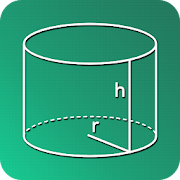# GeometrySoln : Geometry Calculation Solver - Lumos Educational App Store41
Price -fREE
\$0

#### DESCRIPTION:

GeometrySoln is Geometry Calculator for Students and Teachers, which makes Geometry Calculations very Easy and Quick. From Basic Calculation of Perimeter of a Square, to Calculating a Volume of a Cube, you can get it all. It displays the formulas to calculate the same and also it will calculate, the Perimeter, Area, Volume, etc, It will also display a Detailed Calculation Steps on how the result was obtained. You can work with Following Shapes Square Triangle Rectangle Parallelogram Trapezium Quadrilateral Rhombus Polygon Circle Annulus Ellipse Torus Ball Cube Parallelepipe

#### OVERVIEW:

GeometrySoln : Geometry Calculation Solver is a free educational mobile app By .It helps students in grades HS practice the following standards HSG.GMD.A.1.

This page not only allows students and teachers download GeometrySoln : Geometry Calculation Solver but also find engaging Sample Questions, Videos, Pins, Worksheets, Books related to the following topics.

1. HSG.GMD.A.1 : Give an informal argument for the formulas for the circumference of a circle area of a circle volume of a cylinder pyramid and cone. Use dissection arguments, Cavalieris principle, and informal limit arguments..

HS

#### STANDARDS:

HSG.GMD.A.1

Developer:

Software Version: 1.3

Category:

### RELATED APPSEdSearch WebSearch